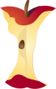Home > Math Topics > Trigonometry >

## Application of the Standard Law of Sines#### Aligned To Common Core Standard:

High School Geometry - HSG-SRT.D.10

### Printable Worksheets And Lessons

• Leg Length Step-by-Step Lesson- Find the leg of a triangle when you are given two angle measures and a side.

• Guided Lesson - These problems all hover around the type of question you saw in the lesson.

• Guided Lesson Explanation - The answers and process all start with the use of Sine equation.

• Practice Worksheet - I focus most of the problems on finding legs of triangles because this is the most common question on the national exams.

• Matching Worksheet - Match the lengths of legs to the missing parts of the triangles that are explained.

###Answer Keys

View Answer Keys- All the answer keys in one file.

###(Click Here to Upgrade)

#### Homework Sheets

You are given some set dimensions and we ask you to find some missing sides and unknown lengths.

#### Practice Worksheets

Some problems will give sides, some will give you angles, and others will give you both.

#### Math Skill Quizzes

We work off of one basic triangle guide to answer these.

 Quiz 1 Quiz 2 Quiz 3